# Field on plan

Plan has a scale of 1: 2500.Determine dimensions in centimeters which will have on plan field with a length of 310 meters and a width of 182.5 meters.

Correct result:

a =  12.4 cm
b =  7.3 cm

#### Solution: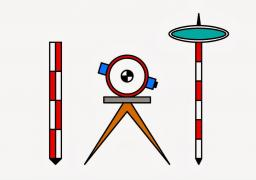We would be pleased if you find an error in the word problem, spelling mistakes, or inaccuracies and send it to us. Thank you!#### You need to know the following knowledge to solve this word math problem:

We encourage you to watch this tutorial video on this math problem:

## Next similar math problems:

• Plan of the villagePlan of the municipality in 1:1000 scale has plotted garden with dimensions 25 mm and 28 mm. Determine the area of gardens in ares in reality.
• Hectares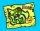Determine the area of the rectangular land (in ha), which has dimensions of 3 cm and 4.5 cm on the plan with a scale of 1: 40,000.
• PlotThe plot on which Mr. Kalous is to build a house has the shape of a rectangle. On a 1: 500 scale, its dimensions are 7cm and 5.5cm. Find out the dimensions of the plot. Calculate the parcel size.
• Map scaleGarden has on plan on a scale of 1: 150 width 22 cm and length 35 cm. What is the real area of the garden?
• Scale of planOn the plan of the village in the scale of 1: 1000 a rectangular garden is drawn. Its dimensions on the plan are 25mm and 28mm. Determine the area of the garden in ares.
• Hřiště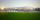Map scale is 1: 5000. The playground is rectangular and on the map has dimensions 10 cm and 5 cm What is area of playground in square meters in reality?
• Map scaleThe rectangular plot has in a scale of 1: 10000 area 3 cm2 on the map. What content does this plot have on a 1:5000 scale map?
• Rounding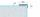What width and length in centimeters may have rectangle land when round the dimensions to the meter , the width is 5 m and length 7 meters?
• FloorThe floor area of ​​the room is 31 m2 and has a width of 4.3 m. How many centimeters of circumference measured floor on the map at the scale 1:75?
• A mapA map with a scale of 1: 5,000 shows a rectangular field with an area of 18 ha. The length of the field is three times its width. The area of the field on the map is 72 cm square. What is the actual length and width of the field?
• Painter 3Dad want to paint wall high 250 cm wide and 230 cm with wallpaper. How many meters must buy wallpaper if wallpaper width is 60 cm?
• Two monuments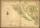The distance between two historical monuments on a map with a scale of 1: 500000 is 48 mm. Find the actual distance between these monuments in km.
• Map scaleOn a 1:1000 scale map is a rectangular land of 4.2 cm and 5.8 cm. What is the area of this land in square meters?
• Rectangular fieldA rectangular field has a diagonal of length 169m. If the length and width are in the ratio 12:5. Find the dimensions of the field, the perimeter of the field and the area of the field.
• Harvester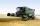Harvester has cutting width 4 m and harvested grain in the field, which is 87 meters wide. Harvester moves along the length of the field for six minutes, going back (without cutting) it takes half the time. Each turn at the end field take one minute. How
• GardenThe rectangular garden has dimensions of 27 m and 30 m. Peter and Katka split it in a ratio of 4:5. How many square meters did Katkin measure part of the garden?
• Square garden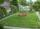On the plan with a scale of 1:1500 is drawn as a square garden with area 81 cm2. How many meters is long garden fence? Determine the actual acreage gardens.